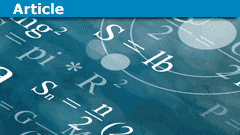# Comments - Friends, strangers, 7825 and computers

• Insights
• micromass
In summary, the conversation discussed a new PF Insights post about a mathematical problem involving the number 7825. The participants also shared their thoughts on famous mathematicians and their contributions, and the role of computers in proving theorems. They also mentioned a reference for further reading.

#### micromass

Staff Emeritus
Homework Helper
micromass submitted a new PF Insights post

Friends, strangers, 7825 and computersContinue reading the Original PF Insights Post.

•ProfuselyQuarky and fresh_42
Nice article Micro!

To start with: It's been a great pleasure to read your Insight. I wonder if Ramsey's theory will share a similar fortune as many other problems that could be formulated so easily.

I'm always surprised again how some numbers or their properties fascinates us. When I think of Pythagoras I usually think of him as an ancient esoteric, a charlatan. On the other hand when it comes to Ramanujan I think of him as one of the greatest, possibly the greatest virtuoso who ever played on the gamut of numbers. Does this discrepancy exist because Ramanujan contributed so much more to number theory than taxicab numbers or am I simply unfair to Pythagoras.

It's also worth reading Simon Singh's book onhttps://www.amazon.com/dp/1841157910/?tag=pfamazon01-20. It brought literally thousands of hobby mathematicians to try a solution because it could be stated so easily. Today it's believed that Fermat never had a real proof of it, or at best only for the case ##n=3.## One must know that at the time, some scholars made fun of it to write their colleagues letters, in which they stated to have proven something without actually given a proof, just to see whether the other one can find out. Nevertheless it was a real booster for number theory. The proof itself, however, is only understood by a handful of mathematicians (meanwhile maybe some more) and requires deep insights into the theory of elliptic curves and (as far as I know) the theory of modular forms. It is agreed to be proven, although only a few could verify this. What makes such a situation different from a computer based handling of cases? I remember I once had a computer proven some cases as well to verify a theorem. Long after it has been accepted, I found some loopholes in the program. It didn't change the result, for I could close them but nobody (except me) ever really noticed. Another prominent example is the Four-Color-Theorem.
As in chess, I think we will have to develop a common ground on which we will judge computer based proofs. Personally I think it will require to say goodbye to some selfish human attitudes.

Back to ##7825 = 5^2 \cdot P_{5\cdot P_{5+1}}##. What fascinates us on numbers? One can probably take any number and construct obscure relations around it. I like the hypothesis that it comes from our evolutionary based need to recognize patterns. It is kind of fascinating by itself that this pattern-recognition led to so many theorems and knowledge in number theory. And that it has such an enormous history from Pythagoras, over Ramanujan to Wiles.

Last edited by a moderator:
Great article!

R(5,5) is between 43 and 49. So we have an example of a group of 42 where no subgroup of 5 exists, and we have a proof that groups of 50 people have to have such a subgroup?

Constructing it with the given recursive rule leads to a higher upper bound:
R(4,3) <= R(3,3) + R(4,2) = 6+4 = 10
R(5,3) <= R(4,3) + R(3,3) = 16
R(4,4) <= R(4,3) + R(3,4) = 20
R(5,4) <= R(4,4) + R(5,3) = 36
R(5,5) <= R(5,4) + R(4,5) = 72

We don’t know what’s special about 7825 that would ruin everything.

Reference https://www.physicsforums.com/insights/friends-strangers-7825-computers/

This isn't like the four-color theorem. This is a computation that doesn't require any second-order logic. Beyond 7825, the induction is trivial for us humans, and we can let the computer halt. With only a finite amount of exact bead-pushing, what would such a question even mean? What could an answer to "why" be, except "I just showed you"?